# Let 4

Let z = 6 + 5i and w = 3 - i.

Compute the following and express your answer in a + bi form.
w + 3z

a =  21
b =  14

### Step-by-step explanation:

$b=14=14$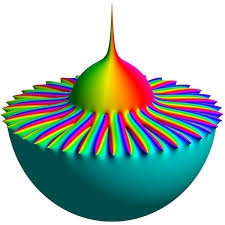Did you find an error or inaccuracy? Feel free to write us. Thank you!Tips to related online calculators

## Related math problems and questions:

• Goniometric form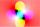Determine the goniometric form of a complex number z = √ 110 +4 i.
• Linear combination of complex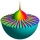If z1=5+3i and z2=4-2i, write the following in the form a+bi a) 4z1+6z2 b) z1*z2
• Evaluate 18Evaluate the expression (-4-7i)-(-6-9i) and write the result in the form a+bi (Real + i* Imaginary).
• Is complexAre these numbers 2i, 4i, 2i + 1, 8i, 2i + 3, 4 + 7i, 8i, 8i + 4, 5i, 6i, 3i complex?
• ABS CNCalculate the absolute value of complex number -15-29i.
• De Moivre's formula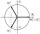There are two distinct complex numbers z, such that z3 is equal to 1 and z is not equal to 1. Calculate the sum of these two numbers.
• Mappings of complex numbers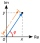Find the images of the following points under mappings: z=3-2j w=2zj+j-1
• Linear imaginary equationGiven that 2(z+i)=i(z+i) "this is z star" Find the value of the complex number z.
• The modulus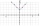Find the modulus of the complex number 2 + 5i
• Distance two imaginary numbsFind the distance between two complex number: z1=(-8+i) and z2=(-1+i).
• Complex number coordinatesWhich coordinates show the location of -2+3i
• Eq2 equationsFor each of the following problems, determine the roots of the equation. Given the roots, sketch the graph and explain how your sketch matches the roots given and the form of the equation: g(x)=36x2-12x+5 h(x)=x2-4x+20 f(x)=4x2-24x+45 p(x)=9x2-36x+40
• Compute 4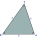Compute the exact value of the triangle area with sides 14 mi, 12 mi, and 12 mi long.
• Cis notationEvaluate multiplication of two complex numbers in cis notation: (6 cis 120°)(4 cis 30°) Write result in cis and Re-Im notation.
• Subtracting complex in polar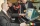Given w =√2(cosine (p/4) + i sine (pi/4) ) and z = 2 (cosine (pi/2) + i sine (pi/2) ), what is w - z expressed in polar form?
• Let z1=x1+y1i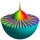Let z1=x1+y1i and z2=x2+y2i Find: a = Im (z1z2) b = Re (z1/z2)
• Im>0?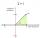Is -10i a positive number?